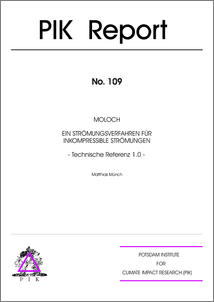Repository: Freie Universität Berlin, Math Department

# MOLOCH - Ein Strömungsverfahren für inkompressible Strömungen - Technische Referenz

Münch, M. (2008) MOLOCH - Ein Strömungsverfahren für inkompressible Strömungen - Technische Referenz. PIK-Report (109).Preview

1MB

## Abstract

Many flows relevant for climate research, weather forecasting, heating, ventilation, air-conditioning (HVAC), and fire safety are characterized by relatively small velocities compared to the speed of sound. The efficient computation of such low Mach number flows requires computational methods that avoid the Courant-Friedrich-Lewy time step restriction, based on the speed of sound. An essential issue in the context meterological flow modelling, as investigated at the Potsdam Institute for Climate Impact Research, is the construction of efficient numerical schemes for such flows that are in conservation form with respect to all physically conserved quantities, i.e., mass, momentum, and energy. Extending initial developments by Klein et. al [9, 13, 15, 19, 20], a new scheme for such flows is currently under development which accounts for flows in three space dimensions, is fully conservative, with second order accuracy and covers the entire regime 0 M 1. To transfer this scheme to practical applications, the author has created the programm code MOLOCH. This technical report collects the background theory of the scheme and describes the current state of its implementation. The code is based on a finite-volume method using a cartesian grid. Currently, the scheme is limited to Zero-Mach number. The first four chapters describe the mathematical background of the scheme. After a short presentation of the underlying set of equations, the result of a one-scale asymptotic analysis is discussed to elucidate the main problems in the context of constructing numerical simulation schemes, and to motivate the construction of the method. Chapter 5 describes the details of the numerical technique and provides additional information regarding the discretization and the implementation of boundary conditions. Chapter 6 describes the implementation of gravitational forces, necessary to run the falling droplet test case, which will be described in Chapter 7. An empirical convergence study confirms the predicted second order accuracy of the implemented scheme. Current work in progress aims at extending the scheme presented here to the meteorologically important class anelastic, instead of incompressible, flow models. The computational code structure as presented here will transfer to these applications without change.

Item Type: Article Mathematical and Computer Sciences > Mathematics > Applied Mathematics Department of Mathematics and Computer Science > Institute of Mathematics > Geophysical Fluid Dynamics Group 649 Ulrike Eickers 29 Jul 2009 14:55 03 Mar 2017 14:40

Repository Staff Only: item control page